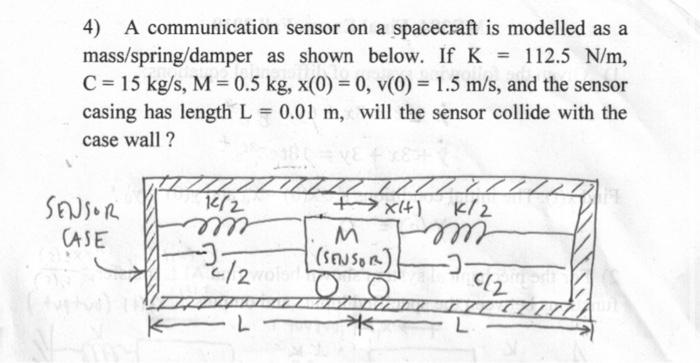Home / Expert Answers / Mechanical Engineering / homework-4-a-communication-sensor-on-a-spacecraft-is-modelled-as-a-mass-spring-damper-as-shown-b-pa827

# (Solved): homework 4) A communication sensor on a spacecraft is modelled as a mass / spring/damper as shown b ...

homework4) A communication sensor on a spacecraft is modelled as a mass spring/damper as shown below. If , , and the sensor casing has length , will the sensor collide with the case wall?

We have an Answer from Expert

The equation of motion for the sensor is:

where:
m is the mass of the sensor (0.5 kg)
c is the damping coefficient (15 kg/s)
k is the spring constant (112.5 N/m)
x is the displacement of the sensor from its equilibrium position
x? is the velocity of the sensor
x¨ is the acceleration of the sensor
The initial conditions are:

We have an Answer from Expert